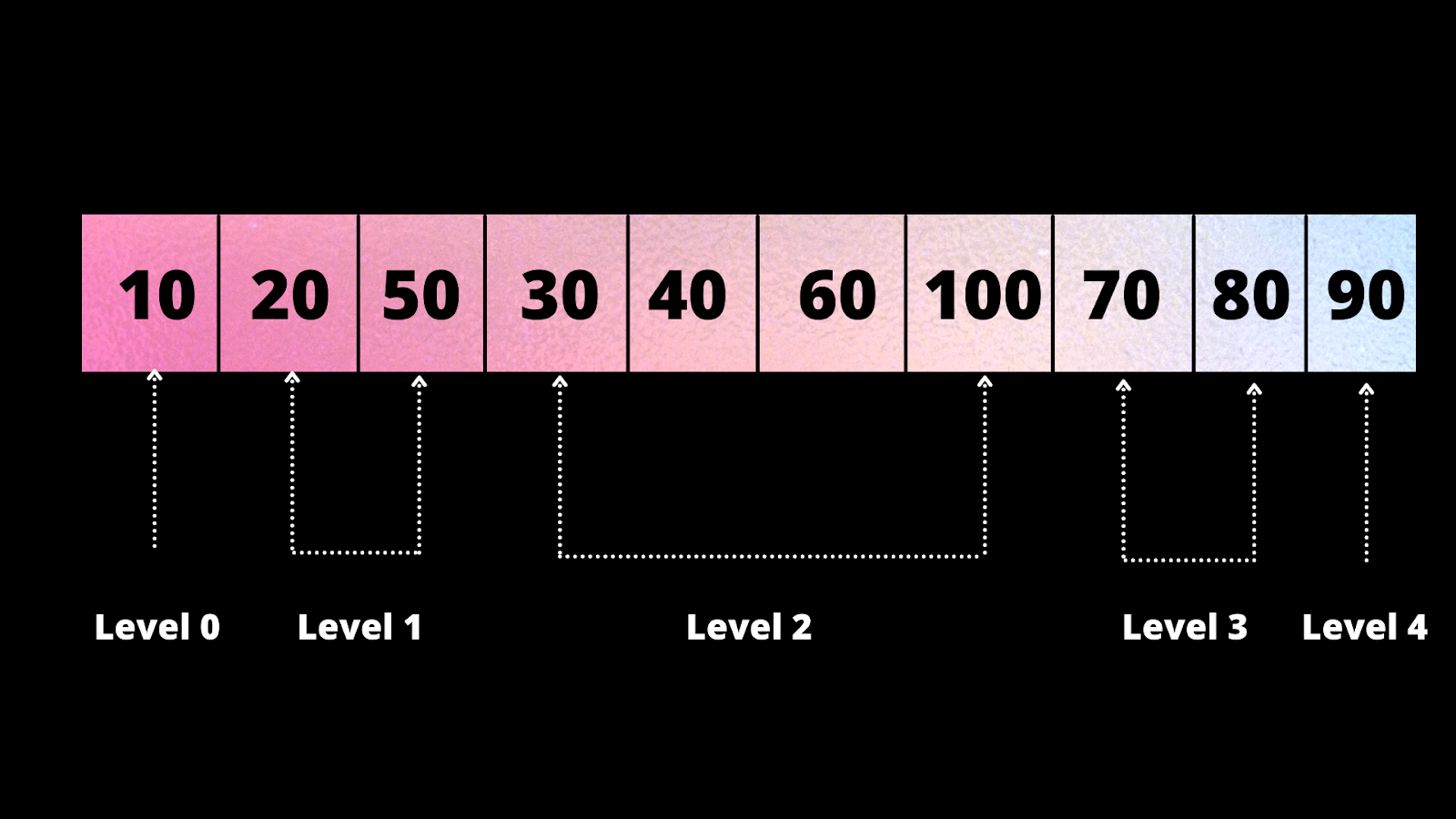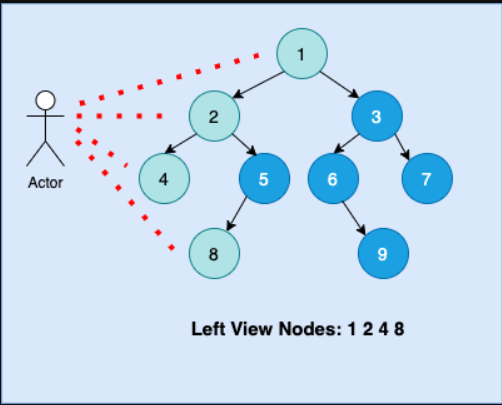# Left View of a Binary Tree in Java

## Introduction

Let’s assume a person is sitting at the left and wishes to view a tree. Nodes would block some of his views when the person’s line of sight would be limited from just one direction. The nodes to be printed are the nodes in the tree visible when viewed from the left side. We need to find the approaches to find the left view of a binary tree.

Let’s see how a tree would be visible to a person wishing to view a tree from the left.

So the nodes visible to a person viewing the tree from the left would be 10, 20, 30, 70, and 90. Left nodes would hide the rest of the nodes. We are asked for a technique to print the left view of the nodes as a result.

This problem can have multiple approaches. Let’s go through them and understand which is the more optimal solution.

## Solving using Level Order Traversal

If we consider the above example, notice the leftmost(first) node in each level of the tree is the one that is considered in the final answer. This approach is also known as an “Iterative Method.”This method can be used to find the left view of a binary tree.

We are given that the tree has five levels; let’s see all the nodes in Level Order from left to right.So, to perform Level Order Traversal, the nodes would be considered level-wise. This means that each node would access the child at each level before moving to the next level.

We have these nodes respective to each level,

Level 0 - 10

Level 1 - 20, 50

Level 2 - 30, 40, 60, 100

Level 3 - 70, 80

Level 4 - 90

Notice the first node in each level is the node that is to be printed for the result.

### Algorithm

For this approach, to find the left view of a binary tree, we will use a queue for the traversal of the tree and check if the elements are present, and print them accordingly to each level of the tree. The approach is known as an iterative approach because, after the traversal of the nodes, a NULL pointer is assigned to mark the end of the level. To do the level order traversal, we then iterate from the first level to the last level and push the children of the nodes in the queue.

Pseudocode:

### Implementation in Java

OUTPUT

Time Complexity: The time complexity of the algorithm to find the left view of a binary tree using level order traversal is O(N) where N is the number of nodes in the Binary Tree.

Space Complexity: The space complexity of the algorithm is O(N).

## Solving using Recursion in Preorder Traversal

We can solve the problem to find the left view of the binary tree using Recursion in a preorder traversal. We can solve this problem using consistent space and time. The thought is to navigate the tree in preorder traversal and keep up with the maximum level visited up until now. If the current level is more than the maximum level called up until now, then, at that point, the current hub is the primary hub of the current level, and we print it and update the last level to the current level.

### Algorithm

For this approach, we will use a maxLevel variable to keep track of the level visited till now. The recursive calls made while visiting nodes, leftView(node.left, level + 1) are called before leftView(node.right, level + 1) to ensure that the left sub-tree for any node of that node is visited before the right subtree. This ensures that the furthest left node is visited at any level before different nodes at that level.Let’s see how we can use this approach to find the left view of a binary tree.

Pseudocode:

### Implementation in Java

``````import java.util.*;

public class Main {

public static class Node {
int data = 0;
Node left = null;
Node right = null;

Node(int data) {
this.data = data;
}
}

static int idx = 0;
static int max_level = 0;
public static Node create_Tree(int[] arr) {
if (idx == arr.length || arr[idx] == -1) {
idx++;
return null;
}

Node node = new Node(arr[idx++]);

node.left = create_Tree(arr);
node.right = create_Tree(arr);

return node;
}

public static void leftView(Node node, int level) {
if (node == null)
return;

if (max_level < level) {
System.out.print(node.data + " ");
max_level = level;
}

leftView(node.left, level + 1);
leftView(node.right, level + 1);
}

public static void viewSet(Node root) {
leftView(root,1);
}

public static void solve() {
int[] arr = { 10, 20, 30, -1, -1, 40, -1, -1, 50, 60, 70, -1, -1, 80, 90, -1, -1, -1, 100, -1, -1 };
System.out.println("Using Recursion Method");
Node root = create_Tree(arr);
viewSet(root);
}

public static void main(String[] args) {
solve();
}
}``````

OUTPUT

Time Complexity: The time complexity for the above algorithm which is used to find the left view of a binary tree is O(N), where N is the number of nodes in the binary tree as the function traverses the tree.

Space Complexity: The space complexity of the algorithm which is used to find the left view of a binary tree is O(H), where H is the height of the binary tree.In case of skewed trees the height of the tree can become N which gives a worst of O(N).

1. What is a Binary Tree?
Ans - A generic tree with at most two child nodes for each parent node is known as a Binary Tree. An empty tree is also considered a valid Binary Tree.

2. What is the left view of a Binary Tree?
Ans - The set of nodes visible when a tree is viewed from the left is known as the left view of a Binary Tree.In the above example, the nodes visible from the left side are 1, 2, 4, and 8.

3. What is the time complexity of Level Order Traversal to view the left view of a binary tree?
Ans - The time complexity of a level order traversal is O(N), where N is the number of vertices in the tree.

4. How can you write an algorithm for the recursion approach for the left view of a binary tree?
Ans - We can explain the algorithm to find the left view of a binary tree using the recursion approach, we will use a maxLevel variable to keep track of the level visited till now. The recursive calls made while visiting nodes, leftView(node.left, level + 1) are called before leftView(node.right, level + 1) to ensure that the left sub-tree for any node of that node is visited before the right subtree. This ensures that the furthest left node is visited at any level before different nodes at that level.

## Key Takeaways

In this blog, we learned the left view of a binary tree and how we can use different approaches to solve the problem.

• The first approach was to use level order traversal and a queue. In this approach, the queue was used for the traversal of the tree and check if the elements are present. The approach is also known as an iterative approach because, after the traversal of the nodes, a NULL pointer is assigned to mark the end of the level. To do the level order traversal, we then iterate from the first level to the last level and push the children of the nodes in the queue.

• The second approach was to use recursion to solve the problem in a preorder traversal. In this approach, we used a maxLevel variable to keep track of the level visited till now. The recursive calls which were made while visiting nodes, leftView(node.left, level + 1) are to be called before leftView(node.right, level + 1) to ensure that for any node, the left sub-tree of that node is visited before the right subtree. This ensures that at any level, the furthest left node is visited before different nodes at that level.

If you want to learn about finding the right view of a binary tree, we have a separate article that covers the approaches used to solve the problem. Do give it a read.

Visit here to learn more about trees. And practice similar problems on CodeStudio. If you liked this blog, share it with your friends.